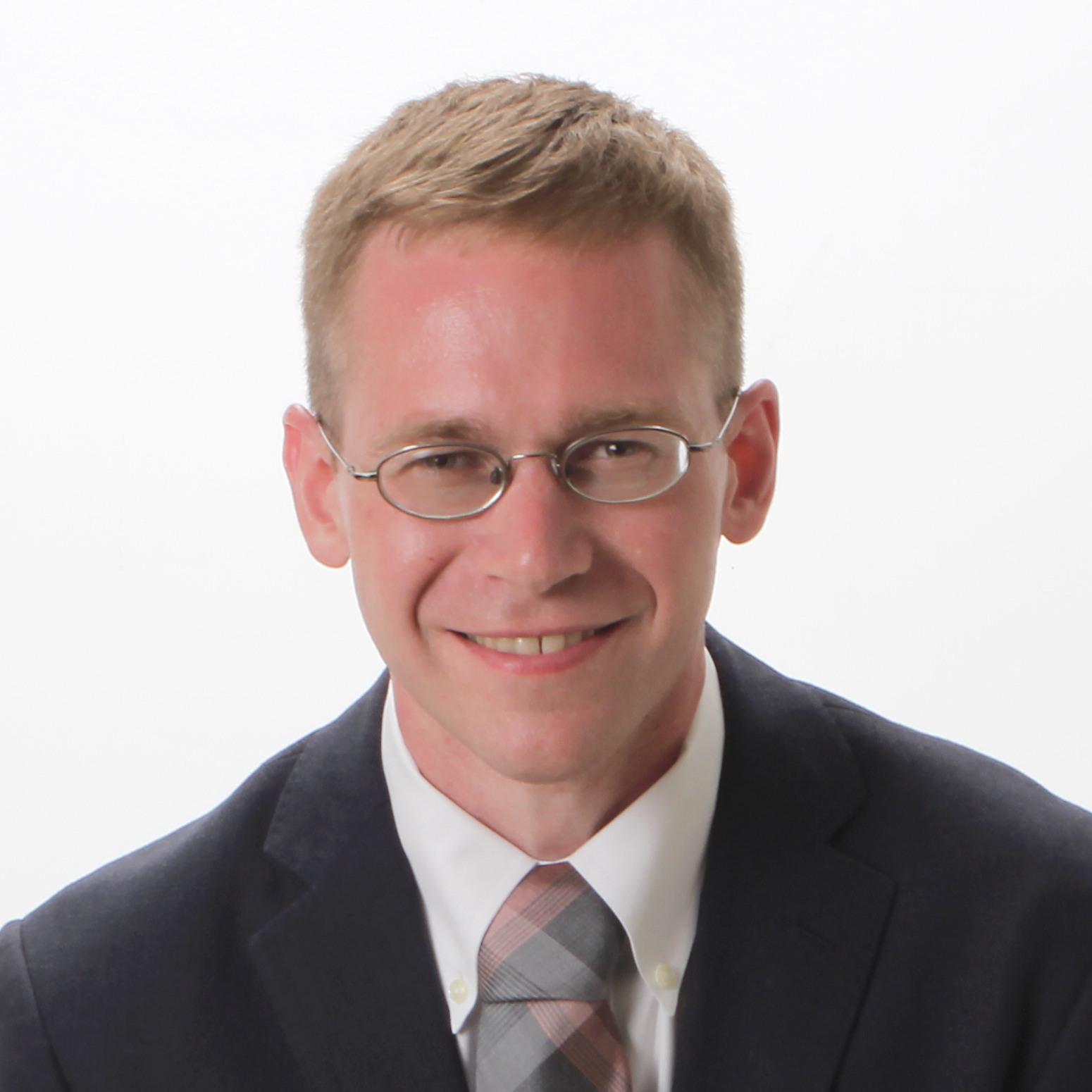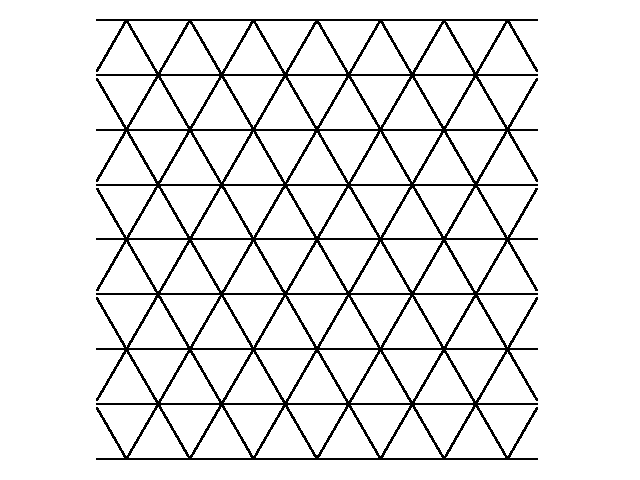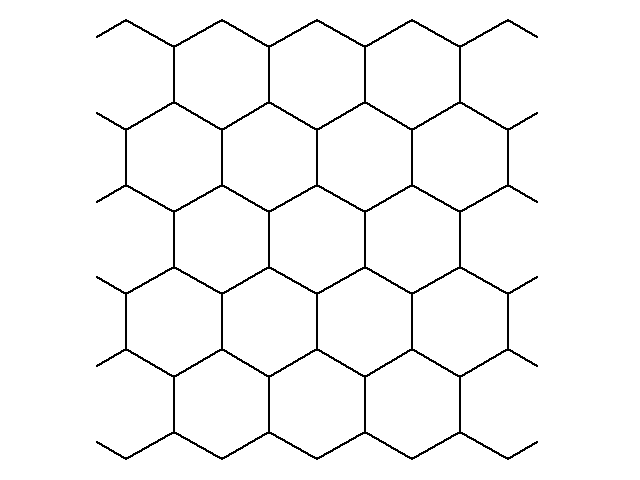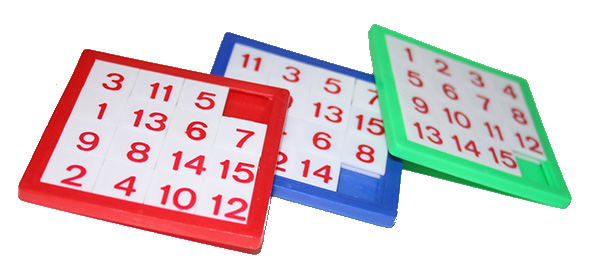Robert D. Hough
Department of Mathematics
Stony Brook University
Stony Brook, NY 11794
e-mail: robert.hough at stonybrook.edu
My cv.

Beginning Fall 2021 I will be Associate Professor of Mathematics at Stony Brook University. My research interests are in probability and analytic number theory. Previously I have been a post-doctoral fellow at the Institute for Advanced Study, Princeton, and at the Mathematical Institute, Oxford and DPMMS, Cambridge. I completed my PhD in Mathematics at Stanford University in 2012. My graduate research was sponsored by a Ric Weiland Graduate Research Fellowship. I have also completed a masters degree in computer science at Stanford, with an emphasis in algorithms. As an undergraduate, I won Stanford's J.E. Wallace Sterling Award for scholastic achievement, and a Boothe Prize for excellence in writing. I was a member of Stanford's Putnam team for three years and scored a perfect score on AMC12. I help Sasha Kirillov to coordinate Stony Brook's Math Circle for high school students, and I run Stony Brook's Putnam Seminar.

I won the 2017 David P. Robbins Prize from the Mathematical Association of America. I've recently won a 2020 Sloan Research Fellowship from the Sloan Foundation, and a 2020 Stony Brook Trustees Faculty Award.

My research is supported by NSF Grants DMS-1712682, "Probabilistic methods in discrete structures and applications," and DMS-1802336, "Analysis of Discrete Structures and Applications."

Analysis seminar: Together with Chris Bishop and Raanan Schul, I am organizing the Stony Brook Analysis Seminar. Further information is available here.

Putnam seminar: I am coordinating the 2020 Putnam Seminar, which meets on Fridays from 6-7:30 pm in the Math Tower Common Room during Fall term, starting Sept. 4. The Putnam exam is February 20, 2021, from 10am-6pm in Math Tower P-131. More information is available here. Last year's website is available here.

Teaching:

In Spring of 2021 I am teaching MAT 308, Differential Equations with Linear Algebra.

In Fall of 2019 I taught Mat 551, Analysis III: Functional Analysis.

In Fall of 2018 I taught Math 608: Topics in analytic number theory. The course combined a brief introduction to analytic number theory with several modern results from prime number theory.

In Spring of 2017 I taught Topics in Probability, which was a fast paced course covering topics including Brownian motion and the Gaussian free field, Stein's method of normal approximation, multiple ergodic averages, and concentration of measure. Slides from the course are available here.

Students:

Areas of research interest:

• Probability, discrete mathematics, analytic number theory

Specific research projects:

• Several years ago I solved an old problem of Erdős by showing that the least modulus of a distinct covering system of congruences cannot be arbitrarily large. The third edition of Richard Guy's book Unsolved problems in number theory writes that Erdős had offered as much as \$1000 for a solution of the problem. The solution used a device from combinatorial probability called the Lovász Local Lemma, together with a fibering argument and a pseudorandom measure. Here are talks by Ben Green, and myself discussing the solution. I have an ongoing research project with Pace Nielsen at BYU studying covering systems. This paper with Pace proves that every distinct covering system of congruences has a modulus divisible by either 2 or 3.

• A number of years ago Persi Diaconis tricked me into studying a random walk on a group, and I have been doing so from time to time ever since. I am especially interested in the cut-off phenomenon, in which a Markov chain transitions to stationarity in a narrow window about its mixing time. These papers use (new) integral formulae for the characters of the symmetric and (with Yunjiang Jiang) orthogonal groups. This preprint with Persi solves an old problem about the mixing time of coordinates in finite nilpotent groups and solves a problem of Emmanuel Breuillard on random walk on the Heisenberg group.

• Compared to central limit theorems, local limit theorems give fine scale information about the limiting distribution of a random walk, since these types of limit theorems hold without rescaling. My recent preprint obtains a general local limit theorem on nilpotent Lie groups which contains a number of prior local limit theorems as special cases. The limit holds for a general measure subject to a moment condition. My paper on cycle walks proves a local limit theorem in Euclidean space which is uniform in the dimension and the number of steps of the walk. A local limit theorem is also used in my recent paper with Dan Jerison and Lionel Levine on abelian sandpiles on the square lattice.

• Hyojeong Son has won the 2018 Summer Math Scholarship, congratulations Hyojeong! Together we've developed code in SciPy to study functions with integral graph Laplacian (functions which are harmonic modulo 1) on several common lattices, including the triangular, honeycomb, face centered cubic, and D4 lattices, determining the spectral gap and asymptotic mixing time. We also studied sandpiles with open and periodic boundary conditions, and have proved a cut-off phenomenon for sandpiles on an arbitrary periodic plane or space tiling. In two dimensions, the asymptotic mixing time is shown to be equal for periodic and open boundary condition, but for the D4 lattice, there is an open boundary condition with which the mixing time is later, and controlled by the 3 dimensional boundary behavior. As part of our work we obtain a method of determining the Green's function of an arbitrary periodic plane or space tiling.Hyojeong presented the research on a poster at the 2019 Joint Math Meetings in Baltimore. An image of her poster is available here. A link to an arXiv preprint of our work is available here. The current version of Hyojeong's thesis is available here.

• Yang Chu has won the 2019 Summer Math Scholarship, congratulations Yang! A discussion of our project has now appeared in Quanta.

An 'n^2-1' puzzle consists of an nxn board with n^2-1 numbered pieces and one empty square. A move in the puzzle consists of sliding one of the numbered pieces into the empty square.Together we've shown that a single numbered piece on the n^2-1 puzzle given periodic boundary conditions has a randomized location after order n^4 random moves, and that if the number of random moves tends to infinity compared to n^4, then the number of pieces left in their original position converges to a Poisson(1) distribution. The distribution of pieces on the board converges to uniform in order n^4 log n random moves. A preprint of our work is available here. Yang's poster from the 2020 Joint Math Meetings is available here. Yang's thesis is available here.

• I have studied several distribution problems in analytic number theory, including the distribution and extreme values of L-functions, and the distribution of shapes of fixed torsion ideal classes in imaginary quadratic fields. In a recent paper, I generalize the Shintani zeta function to an object giving spectral information about the distribution of the shapes of cubic orders. In two recent preprints I refine this to obtain a spectral decomposition of the shape of cubic fields, and a spectral decomposition of the joint distribution of the shape of a quartic field and its cubic resolvent ring. When the spectral object is a cusp form, it is shown that the resulting zeta function is entire. This is part of an ongoing project with Frank Thorne at USC and Takashi Taniguchi at Kobe University. Recently, I have obtained an exact formula for the local condition of maximality in quartic rings. The cubic analogue of this result played a key role in Taniguchi and Thorne's proof of a secondary main term in the count of cubic fields. Joint with Eun Hye Lee preprint I've calculated the Eisenstein spectrum in the shape of cubic rings, which calculates the missing mass in the distribution of their shape as the pole of the Dirichlet series, answering a question that was raised at the American Institute of Math. In this preprint we show that the Shintani zeta function is subconvex. Our method is general, and in work in progress we are able to prove that the zeta function of a general prehomogeneous vector space is subconvex. These give among the largest degree examples of zeta functions which are subconvex, aside from those which are built from products.

Slides from my talk on fields at PANTS XXXIII.

Slides on mixing and sandpiles, from a talk at the University of Washington probability seminar.

A recording of my talk at CANT2020 on the 15 puzzle.

Slides from a talk at University of South Carolina on analytic properties of zeta functions of prehomogeneous vector spaces.

An illustration of Leon Green's Theorem.
The right figure is an orbit on the Heisenberg nilmanifold and the left is the projected orbit on the abelianization. Green's Theorem states that the first orbit is asymptotically equidistributed if and only if the second one is.

Publications and preprints:

1. with Eun Hye Lee. Subconvexity of Shintani's zeta functions. Submitted to Trans. AMS. Preprint.

2. with Owen Mireles-Briones. The local limit theorem in high dimensions. (In progress)

3. with Eun Hye Lee. Lower order terms in the shape of cubic fields. (In progress)

4. with Eun Hye Lee. Eisenstein series twisted Shintani zeta functions. Submitted. Preprint.

5. by Marcus Woo. Mathematicians calculate how randomness creeps in. Quanta, 2019. Article. This is a discussion of my project with Yang Chu on the 15 puzzle.

6. with Yang Chu. Randomizing a '15 puzzle'. Preprint.

7. with Hyojeong Son. The spectrum of the abelian sandpile model, Mathematics of Computation. Preprint.

8. with Hyojeong Son. Cut-off for sandpiles on tiling graphs, Annals of Probability, to appear. Preprint.

9. The local zeta function in enumerating quartic fields. Journal of Number Theory. Link. Supporting Mathematica notebooks are available here.

10. The local limit theorem on nilpotent Lie groups. Probability Theory and Related Fields, 2019. Link.

11. The shape of quartic fields. Preprint.

12. The shape of cubic fields. Research in the Mathematical Sciences, 2019. Link.

13. with P. Nielsen. Covering systems with restricted divisibility. Duke Math Journal, 2019. Link.

14. with D. Jerison and L. Levine. Sandpiles on the square lattice. Communications in Math. Physics, 2019. Link.

15. Maass form twisted Shintani L-functions. Proc. AMS 145.10 (2017): 4161-4174. Link.

16. Mixing and cut-off in cycle walks. Electronic Journal of Probability, 22, no.90 (2017): 1-49. Link.

17. with P. Diaconis. Random walk on unipotent matrix groups. Annales scientifiques de l'école normale supérieure, 54(3) 2021: 587-625. Preprint.

18. with Y. Jiang. Asymptotic mixing time analysis of a random walk on the orthogonal group. Annals of Probability, 2017. Link.

19. The angle of large values of L-functions. Journal of Number Theory, 167 (2016): 353-393. Link.

20. The random k-cycle walk on the symmetric group. Probability Theory and Related Fields 165, no. 1 (2016): 447-482. Link.

21. Solution of the minimum modulus problem for covering systems. Annals of Math 181, no. 1 (2015): 361-382. Link.

22. The distribution of the logarithm of orthogonal and symplectic L-functions. Forum Math 26, no. 2 (2014): 523-546. Link. Errata.

23. Zero-density estimate for modular form L-functions in weight aspect. Acta Arith. 154 (2012), 187-216. Link.

24. The resonance method for large character sums. Mathematika 59, no. 01 (2013): 87-118. Link.

25. Equidistribution of bounded torsion CM points. Journal d'Analyse Math, 2019. Link.

26. Summation of a random multiplicative function on numbers having few prime factors. Math. Proc. Camb. Phil. Soc., 150 (2011), pp. 193-214. Link .

27. Tesselation of a triangle by repeated barycentric subdivision. Elec. Comm. Prob., 14 (2009). Link .

Any opinions, findings, and conclusions or recommendations expressed in this material are those of the author(s) and do not necessarily reflect the views of the National Science Foundation.